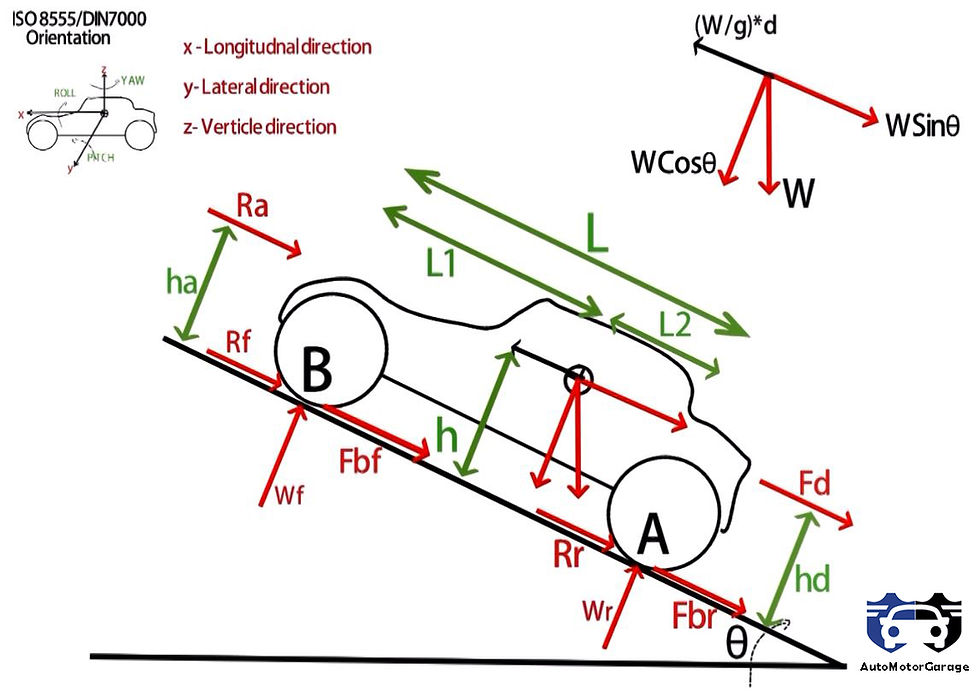top of page
Search

# What is the maximum value of braking force ?

Brakes are the important part of vehicle,without brakes you can't stop your car. While braking rolling resistance of the tire supports the braking force. When you press the brake pedal then brakes produces the opposite torque which pushes the ground in forward direction and ground applies the backward force on the tire to stop the vehicle.Below is the derivation of maximum value of braking force when the brakes are applied.This derivation follows the ISO8555/DIN7000 coordinate system.

## Derivation

### Considering below figure for derivation of maximum braking force !

Assumption

• Car is going up hill

• Ground is making a small angle θ with the horizontal

• Cosθ = 1

• R= fr * W ( R- TOTAL ROLLING RESISTANCE)

• h=ha=hdBalancing all forces Parallel to ground we get:-

(W/g)*d = Fbf+Fbr+Ra+R+WSinθ+Fd (1)

Where, Fbf- front wheel braking force

Fr- rear wheel braking force

Ra- aerodynamic force

R- total rolling resistance(R=Rf+Rr)

Fd- dragging or pulling force(if car is pulling a cart or trailer)

here ,Fd=0

Taking moment about point A we get:-

Wf*L+Ra*h+WSinθ*h-(W/g)*d*h - WCosθ*L2=0

Wf=(W*L2-Ra*h-WSinθ*h+(W/g)*d*h)/L (2)

Taking moment about point B we get:-

Wr=(W*L1+Ra*h+WSinθ*h-(W/g)*d*h)/L (3)

Solving 1,2 & 3 we get:-

Wf=L2*W/L + h/L[Fb+fr*W] (Load on front tire while braking ) (4)

Wr=L1*W/L - h/L[Fb+fr*W] (Load on rear tire while braking) (5)

where , Fb= Fbr +Fbf R=Rf+Rr=fr*W

Maximum braking force:-

Fbf(max)=μ*Wf Fbr(max)=μ*Wr

## Conclusion

At time of braking the weight shifts forward as you can see from the equation 4 & 5 that Wf is greater than Wr. Fb is the total braking force on front and rear tire.

Maximum braking force:-

Fbf(max)=μ*Wf

Fbr(max)=μ*Wr